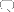# PRTG Manual: Calculating Percentiles

Wikipedia describes a percentile as the value of a variable below which a certain percent of observations fall. Providers often use it in their billing models, e.g. when determining the used bandwidth. With percentile calculation, you can cut off an x percent of peak values from an amount of values.

In PRTG, you can calculate percentiles when creating general reports (see Reports section) or creating historic data reports of a certain sensor (see Historic Data Reports section). If you activate the Percentile Results option in your reports' settings, the according values will be added to the tables. You can customize the following settings:

• Percentile: Enter the percentile number you want to calculate. If you choose, for example, to calculate the 95th percentile, enter "95" here and 5 % of peak values will be discarded.
• Percentile Average: This is the averaging interval in seconds, which is used for percentile calculation. Default value is 300 which is equivalent to 5 minutes.

Percentile Mode: Choose between Discrete and Continuous. Continuous percentile interpolates between discrete values, whereas discrete percentile chooses the next smaller discrete value.

• Discrete percentile means that the value must be a member of the data set. For this kind of calculation you require a discrete distribution. The median of a discrete distribution can not be defined, as such, meaning that the 50th discrete percentile may not necessarily be the median if the value does not belong to an odd number of measurements. Discrete percentiles, as such, should not be used for billing applications.
• Continuous percentile basically means that the measurements are treated as a statistical population and the value is determined by interpolating a value when it isn't present. This means that values are interpolated between actual measurements that are varying around the "perfect" center of the measurements.

More

Knowledge Base: What are percentiles?

Continue

Keywords: Percentile,Discrete (Percentiles),Continuous (Percentiles)

Help
Need More Help?

Go to the Help Center.FEEDBACK Click here to send feedback to Paessler